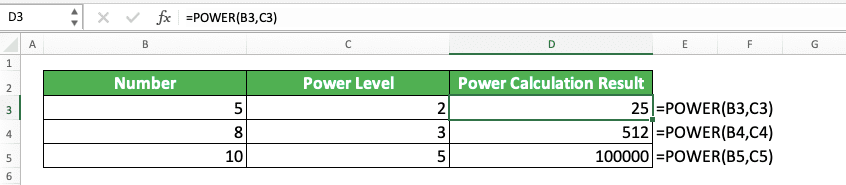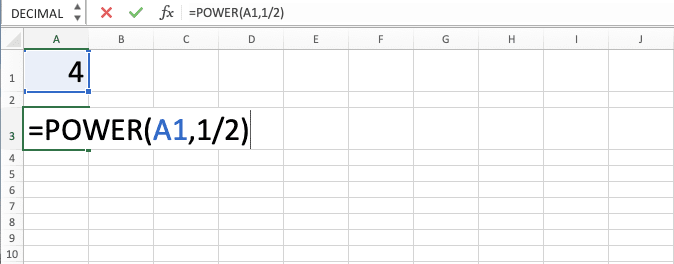How to Use the POWER Formula in Excel: Functions, Examples, and Writing Steps - Compute Expert

# How to Use the POWER Formula in Excel: Functions, Examples, and Writing Steps

Home >> Excel Tutorials from Compute Expert >> Excel Formulas List >> How to Use the POWER Formula in Excel: Functions, Examples, and Writing Steps

In this tutorial, you will learn how to use the POWER formula in excel completely.

When working with numbers in excel, we sometimes need to calculate their power for various purposes. If we use POWER to do the calculation, we can do it easily and fast.

Disclaimer: This post may contain affiliate links from which we earn commission from qualifying purchases/actions at no additional cost for you. Learn more

## What is the POWER Formula in Excel?

POWER is an excel formula you can use to power a number. You just need to input the number you want to power and the power level to get your power calculation result.

## POWER Function in Excel

You can use POWER to get the power calculation result of your number.

## POWER Result

The POWER result is a number which is the power calculation result of the number you input into it.

## Excel Version from Which We Can Start Using POWER

You can start using POWER in excel since excel 2003.

## The Way to Write It and Its Inputs

Here is the general writing form of POWER in excel.

= POWER ( number , power_level )

And here is a bit explanation for each input you need to give when you write POWER in excel.
• number = the number you want to power
• power_level = the level of the power calculation you want to do to your number

## Example of Its Usage and Result

Here is an implementation example of the POWER formula in excel.As you can see here, we just need to input our number and the power level we want to POWER. Do that and we will get the power calculation result of our number immediately!

## Writing Steps

Here is the step-by-step instruction you can use as your guide to write the POWER formula in excel.

1. Type an equal sign ( = ) in the cell where you want to put the power calculation result of your number2. Type POWER (can be with large and small letters) and an open bracket sign after =3. Input the number you want to power or the cell coordinate of the number. Then, type a comma sign ( , )4. Input the power level you want for the power calculation or the cell coordinate where the power level is5. Type a close bracket sign6. Type Enter
7. Done!## POWER Alternative: The Caret Symbol ( ^ )

Besides using POWER, you can also use the caret symbol ( ^ ) to calculate the power of your number in excel. Just type the number you want to power, a caret symbol, and the power level you want for the calculation.

Here is the general writing form which summarizes that explanation.

= number ^ power_level

And here is the implementation example of the caret symbol method to calculate the power of some numbers.We use the same set of numbers we used in the POWER implementation example. If you compare these two examples, you can see that we get the same power results from both methods.

So, if you need an alternative to the POWER formula in excel, just type in a caret symbol from your keyboard!

## Exercise

After you have understood how to use the POWER formula in excel, let’s do an exercise to sharpen your understanding.

### Instructions

Use POWER to do each of the instructions below!
1. Use the power level of 3 to calculate the power of the number at the top!
2. Use the power level of 5 to calculate the power of the number at the top!
3. Calculate the root level of 5 of the number at the top (read the additional note part if you don’t know how to calculate root by using POWER)!

You can also calculate the root of a number in excel by using this POWER formula. The method to do that is by inputting 1/x as the power level of your POWER. The x here is the root level you want for the root calculation process of your number.We can do that because root is the opposite of power in a number calculation process.

Related tutorials you should learn from:

Get updated excel info from Compute Expert by registering your email. It's free!